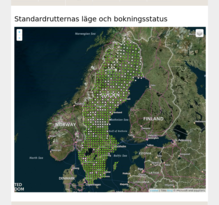# Coordinate Diffusion

About lowering the resolution of spatial point data

recraft@pm.me https://recraft.me (Recraft.ME OÜ)https://github.com/recraft
2018-11-12

Assume we have a leaflet map published with interactivity on the web, that has coordinate point features on it. Below is a low res screenshot of a website with such a map with bird inventory sites data in Sweden.# Scraping coordinates

We can create a function to parse the leaflet features into a fairly tidy data frame. Lets look at the first ten rows of such parsed data.


# for parsing
library(rvest)
library(httr)
library(jsonlite)
library(tibble)
library(dplyr)
library(stringr)

# for geotransform and visuals
library(sp)
library(raster)
library(raster)
library(leaflet)

parse_leaflet_coords <- function(url) {

# get page content from url
www <- url %>% GET %>% content(as = "text")

# pick out features data used by leaflet
tf <- tempfile()
write_lines(www, tf)
cmd <- paste("grep -o -e '\"features\":[[]{.*}}[]]'", tf)

# convert to df
json <- fromJSON(paste0("{", system(cmd, intern = TRUE), "}"),
flatten = TRUE)

as_tibble(json$features) } # parse leaflet coordinates for the routes_night url df <- parse_leaflet_coords(routes_std) # tidy the data to allow for easier plotting p <- df %>% mutate(is_booked = ifelse(is.na(str_match(html, "green")), FALSE, TRUE)) %>% mutate(grid_code = str_extract(label, "\\w{5}")) %>% mutate(grid_desc = str_replace(label, "\\w{5}\\s+(\\w+)", "\\1")) %>% mutate(color = ifelse(is_booked, "darkgreen", "white")) %>% dplyr::select(grid_code, grid_desc, popup, lon, lat, is_booked, color) %>% arrange(lon, lat) paged_table(p %>% dplyr::select(-popup) %>% slice(1:10)) # Fitting a grid to the points We now have the coordinates so we can create spatial points and attempt to find a spatial grid that fits the points given a certain not necessarily modest tolerance for deviations.  # leaflet uses EPSG:4326 so we create spatial points based on the data # and fit points to a grid points <- SpatialPointsDataFrame( data.frame(p$lon, p$lat), data = p, proj4string = CRS("+init=epsg:4326") ) gt <- points2grid(points, tolerance = 0.90) sg <- SpatialGrid( grid = gt, proj4string = CRS("+init=epsg:4326") ) # convert spatial grid to polygons for display in leaflet spdf <- as(sg, "SpatialPolygons") m <- leaflet() %>% addProviderTiles("Stamen.Watercolor", options = providerTileOptions(opacity = 0.25)) %>% addPolygons(data = spdf, weight = 1, color = "darkgray") %>% addCircles(lng = points$lon, lat = points$lat, radius = 1, #stroke = FALSE, color = p$color,
fill = p$color, opacity = 1, popup = p$popup,
m